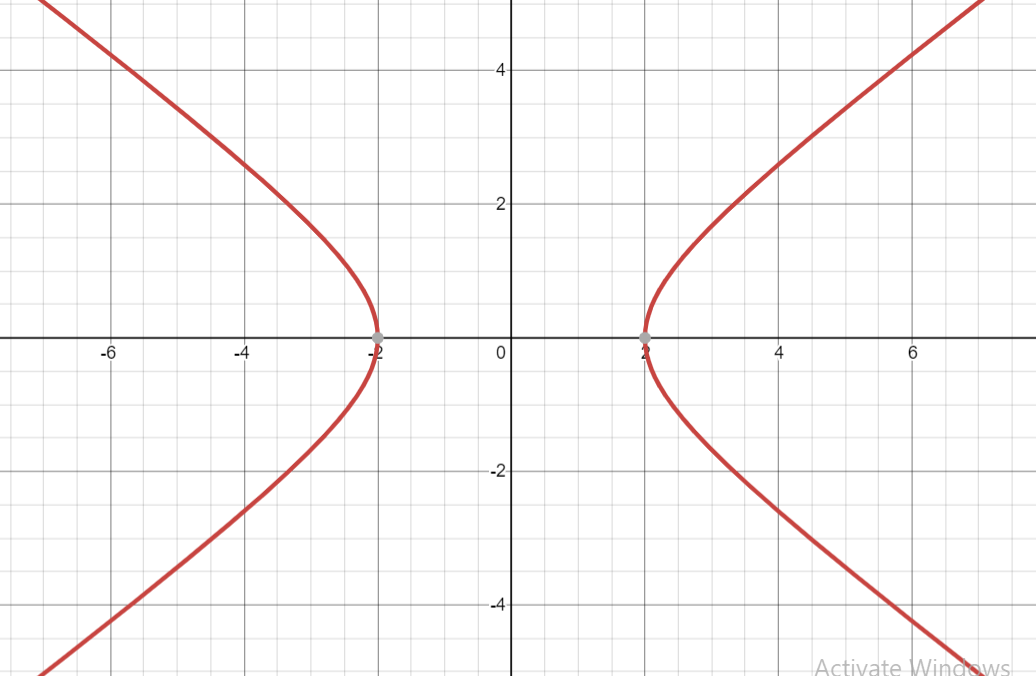QUESTION

# Find the equation of the hyperbola whose conjugate axis is along the y-axis and having length 3 units and distance between foci is 5.$(a){\text{ }}\dfrac{{4{x^2}}}{9} - \dfrac{{{y^2}}}{4} = 1 \\ (b){\text{ }}\dfrac{{{x^2}}}{4} - \dfrac{{4{y^2}}}{9} = 1 \\ (c){\text{ }}\dfrac{{{x^2}}}{4} - \dfrac{{{y^2}}}{9} = 1 \\ (d){\text{ 4}}{{\text{x}}^2}{\text{ - 36}}{{\text{y}}^2}{\text{ = 9 }} \\$

Hint:In this question start with using the general equation of hyperbola which is $\dfrac{{{x^2}}}{{{a^2}}} - \dfrac{{{y^2}}}{{{b^2}}} = 1$, where a and b is half of the length of hyperbola on transverse and conjugate axis, try to find the value of a and b using the fact that distance between foci is 2ae where e is the eccentricity and after finding values of $a$ and $b$ substitute in general equation of hyperbola.

Solution stats here Let the standard equation of hyperbola is
$\dfrac{{{x^2}}}{{{a^2}}} - \dfrac{{{y^2}}}{{{b^2}}} = 1$................................ (1)
Where (a) and (b) are along the transverse and conjugate axis (i.e. along x-axis and y-axis) and it is half of the length of the transverse axis and conjugate axis.
Now it is given that hyperbola has conjugate axis along y axis and has length 3 units.
Therefore our assumption is right,
And 2b = 3.
$\Rightarrow b = \dfrac{3}{2}$......................... (2)
Now it is given that the distance between the foci is 5.
Now as we know distance between foci = 2ae, where (e is the eccentricity of hyperbola and always greater than 1.)
$\Rightarrow 2ae = 5$
$\Rightarrow ae = \dfrac{5}{2}$................................. (3)
Now as we know a, b and e are related as in hyperbola
$\Rightarrow {b^2} = {a^2}{e^2} - {a^2}$
Now from equation (2) and (3) we have,
$\Rightarrow {\left( {\dfrac{3}{2}} \right)^2} = {\left( {\dfrac{5}{2}} \right)^2} - {a^2}$
$\Rightarrow {a^2} = \dfrac{{25}}{4} - \dfrac{9}{4} = \dfrac{{16}}{4} = 4 = {2^2}$
$\Rightarrow a = 2$

Now from equation (1) we have,
$\Rightarrow \dfrac{{{x^2}}}{{{a^2}}} - \dfrac{{{y^2}}}{{{b^2}}} = 1$
Now substitute the values of a and b we have,

$\Rightarrow \dfrac{{{x^2}}}{4} - \dfrac{{{y^2}}}{{{{\left( {\dfrac{3}{2}} \right)}^2}}} = 1$
Now simplify it we have,
$\Rightarrow \dfrac{{{x^2}}}{4} - \dfrac{{4{y^2}}}{9} = 1$
So this is the required equation of hyperbola.

Note – Hyperbola is a symmetrical open curve formed by intersection of a circular cone with a plane at a smaller angle with the axis than the side of the cone. It’s important to see the diagrammatic representation of the equation of the hyperbola obtained as it will help in solving problems of this kind. The graph for hyperbola $\dfrac{{{x^2}}}{4} - \dfrac{{4{y^2}}}{9} = 1$, looks like